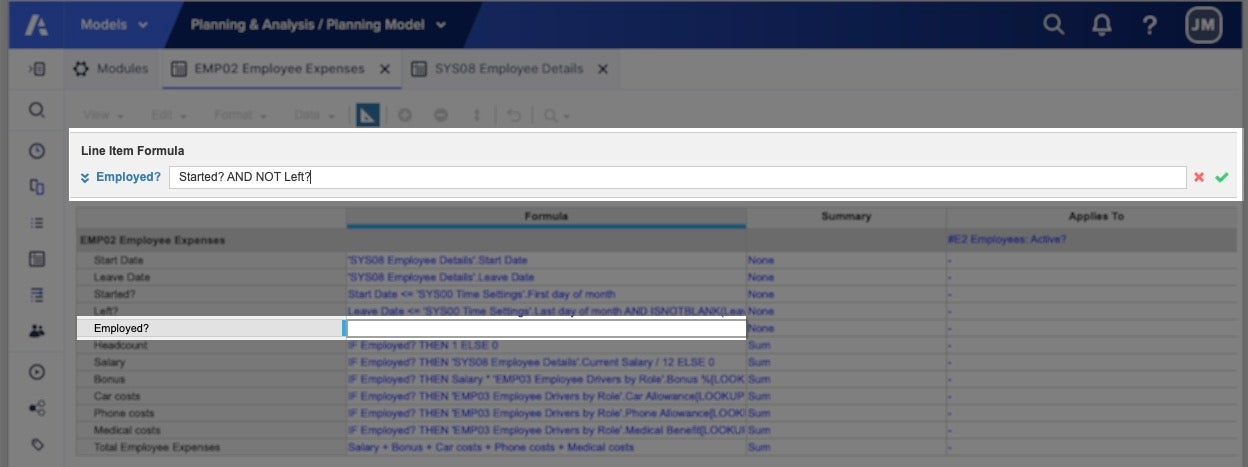The formula bar is best for writing short and simple formulas. To write more complex formulas, use the formula editor.

Always format a line item before entering a formula. The results of the formula must match the format of the cell. For example, if the formula results in a date, the cell must have a date format. The formula is rejected if the formats do not match.

To find information on functions, operators, and how to write formulas, see All functions.

To add a formula using the formula bar:

1.  In Blueprint view, select the line item row. In the formula bar above the line items, the line item name appears next to the editing field.
2. Double-click the formula bar. When the formula bar is activated, the Cancel and OK icons appear to the right of the formula editing bar.1. Enter the formula:
• Type functions and operators directly into the formula bar.
• To add references to line items in the same module, select the line item. The line item reference is automatically added to the formula.
• To add references to line items in different modules, open the modules that you want to refer to and select the line items. The formula is added to the formula editor, which opens at the bottom of the window. The formula editor is fixed when you switch between modules.
2. Select the OK icon to add the formula. Select the Cancel icon to clear the formula.
3. Specify which versions of the model the formula applies to in the Formula Scope column. The Formula Scope option defaults to All Versions